Courses

# Orbital Angular Momentum, Hydrogen Atom (Part - 1) - Angular Momentum, CSIR-NET Physical Sciences Physics Notes | EduRev

## Physics for IIT JAM, UGC - NET, CSIR NET

Created by: Akhilesh Thakur

## Physics : Orbital Angular Momentum, Hydrogen Atom (Part - 1) - Angular Momentum, CSIR-NET Physical Sciences Physics Notes | EduRev

The document Orbital Angular Momentum, Hydrogen Atom (Part - 1) - Angular Momentum, CSIR-NET Physical Sciences Physics Notes | EduRev is a part of the Physics Course Physics for IIT JAM, UGC - NET, CSIR NET.
All you need of Physics at this link: Physics

Introduction

Angular momentum plays a central role in both classical and quantum mechanics. In classical mechanics, all isolated systems conserve angular momentum (as well as energy and linear momentum); this fact reduces considerably the amount of work required in calculating tra jectories of planets, rotation of rigid bodies, and many more.

Similarly, in quantum mechanics, angular momentum plays a central role in understanding the structure of atoms, as well as other quantum problems that involve rotational symmetry.

Like other observable quantities, angular momentum is described in QM by an operator. This is in fact a vector operator, similar to momentum operator. However, as we will shortly see, contrary to the linear momentum operator, the three components of the angular momentum operator do not commute.
In QM, there are several angular momentum operators: the total angular momentum (usually denoted by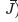, the orbital angular momentum (usually denoted by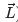) and the intrinsic, or spin angular momentum (denoted by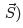. This last one (spin) has no classical analogue. Confusingly, the term “angular momentum” can refer to either the total angular momentum, or to the orbital angular momentum.
The classical deﬁnition of the orbital angular momentum,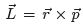can be carried directly to QM by reinterpreting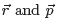as the operators associated with the position and the linear momentum.

The spin operator, S, represents another type of angular momentum, associated with “intrinsic rotation” of a particle around an axis; Spin is an intrinsic property of a particle (nearly all elementary particles have spin), that is unrelated to its spatial motion. The existence of spin angular momentum is inferred from experiments, such as the Stern-Gerlach experiment, in which particles are observed to possess angular momentum that cannot be accounted for by orbital angular momentum alone.

The total angular momentum, J, combines both the spin and orbital angular momentum of a particle (or a system), namely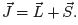Orbital angular momentum

Consider a particle of mass m, momentum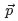and position vector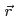(with respect to a ﬁxed origin,= 0). In classical mechanics, the particle’s orbital angular momentum is given by a vector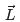, deﬁned by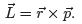. (1)

This vector points in a direction that is perpendicular to the plane containing, and has a magnitude L = rp sin α, where α is the angle between. In Cartesian coordinates, the components ofare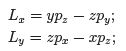(2)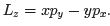The corresponding QM operators representing Lx, Land Lz are obtained by replacing x, y, z and px, py and pz with the corresponding QM operators, giving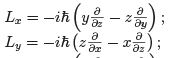(3)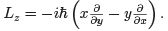In a more compact form, this can be written as a vector operator,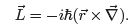(4)

It is easy to verify thatis Hermitian.
Using the commutation relations derived for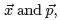, the commutation relations between the diﬀerent components ofare readily derived. For example: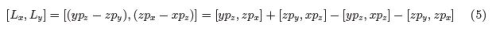Since y and px commute with each other and with z and pz , the ﬁrst term reads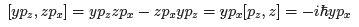(6)

Similarly, the second commutator gives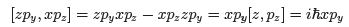(7)

The third and forth commutators vanish; we thus ﬁnd that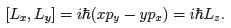(8)

In a similar way, it is straightforward to show that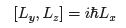(9)

and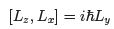(10)

The three equations are equivalent to the vectorial commutation relation: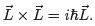(11)

Note that this can only be true for operators; since, for regular vectors, clearly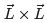= 0.

The fact that the operators representing the diﬀerent components of the angular momentum do not commute, implies that it is impossible to obtain deﬁnite values for all component of the angular momentum when measured simultaneously. This means that if the system is in eigenstate of one component of the angular momentum, it will in general not be an eigenstate of either of the other two components.
We deﬁne the operator representing the square of the magnitude of the orbital angular momentum by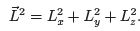(12)

It is easy to show that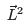does commute with each of the three components: Lx, Ly or Lz .
For example (using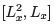= 0):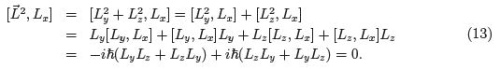Similarly,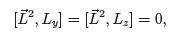(14)

which can be summarized as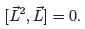(15)

Physically, this means that one can ﬁnd simultaneous eigenfunctions ofand one of the components of, implying that both the magnitude of the angular momentum and one of its components can be precisely determined. Once these are known, they fully specify the angular momentum.
In order to obtain the eigenvalues ofand one of the components of(typically, Lz ), it is convenient to express the angular momentum operators in spherical polar coordinates: r, θ, φ, rather than the Cartesian coordinates x, y, z . The spherical coordinates are related to the Cartesian ones via

x = r sin θ cos φ;
y = r sin θ sin φ;
z = r cos θ.                                              (16)

After some algebra, one gets: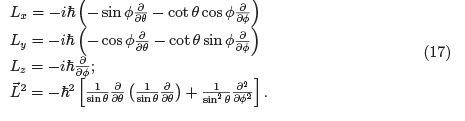We thus ﬁnd that the operators Lx, Ly , Lz anddepend on θ and φ only, that is they are independent on the radial coordinate. All these operators therefore commute with any function of r,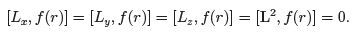(18)

Also, obviously, if a wavefunction depends only on r (but not on θ, φ) it can be simultaneously an eigenfunction of Lx, Ly , Lz and L2. In all cases, the corresponding eigenvalue will be 0. (This is the only exception to the rule that that eigenvalues of one component (e.g., Lx) cannot be simultaneously eigenfunctions of the two other components of L).

Eigenvalues and eigenfunctions of L2 and L

Let us ﬁnd now the common eigenfunctions to L2 and Lz , for a single particle. The choice of Lz (rather than, e.g., Lx) is motivated by the simpler expression (see Equation 17).

Eigenvalues of Lz

Since, in spherical coordinates Lz depends only on φ, we can denote its eigenvalue by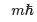and the corresponding eigenfunctions by Φm(φ). We thus have: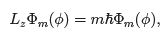(19)
namely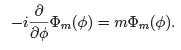(20)
The solutions to this equation are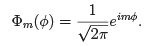(21)

This is satisﬁed for any value of m; however, physically we require the wave function to be single valued (alternatively: continuous), namely Φm(2π) = Φm(0), from which we ﬁnd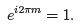(22)

This equation is satisﬁed for m = 0, ±1, ±2, ±3, .... The eigenvalues of the operator Lz are thus m~, with m being integer (positive or negative) or zero. The number m is called the magnetic quantum number, due to the role it plays in the motion of charged particles in magnetic ﬁelds.
This means, that when measuring the z-component of an orbital angular momentum, one can only obtain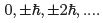Since the choice of the z direction was arbitrary, we see that the component of the orbital angular momentum about any axis is quantized.
The wavefunctions Φm(φ) are orthonormal, namely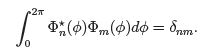(23)

Furthermore, they form a complete set, namely every function f (φ) can be written as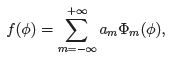(24)
where the coeﬃcients aare C-numbers.

Offer running on EduRev: Apply code STAYHOME200 to get INR 200 off on our premium plan EduRev Infinity!

159 docs

,

,

,

,

,

,

,

,

,

,

,

,

,

,

,

,

,

,

,

,

,

,

,

,

,

,

,

;